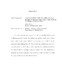## Calculations for the etale fundamental group of curves of genus one or twoThe \'etale fundamental group $\pi_{1, \text{\'et}}(C)$ is a profinite group which classifies covers of algebraic-geometric objects. Its abelianizaton classifies torsors over $C$ with a finite, abelian structure group. When $C$ is a curve over a number field $K$, $\pi_{1, \text{\'et}}^{\textsf{ab}}(C)$ possesses a finite subgroup $\Ker(C/K)$ which records which abelian \'etale covers of $C$ come from geometry. In this thesis multiple algorithms for calculating $\Ker(E/K)$ are presented when $E$ is an elliptic curve over a number field. We indicate how to generalize one of these algorithms to the Jacobian of a genus two curve $C$, allowing for progress to be made on calculating $\Ker(C/K)$.